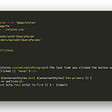# Merge Sorted Array🐧

`Input: nums1 = [1,2,3,0,0,0], m = 3, nums2 = [2,5,6], n = 3Output: [1,2,2,3,5,6]Explanation: The arrays we are merging are [1,2,3] and [2,5,6].The result of the merge is [1,2,2,3,5,6] with the underlined elements coming from nums1.`
`Input: nums1 = , m = 1, nums2 = [], n = 0Output: Explanation: The arrays we are merging are  and [].The result of the merge is .`
`Input: nums1 = , m = 0, nums2 = , n = 1Output: Explanation: The arrays we are merging are [] and .The result of the merge is .Note that because m = 0, there are no elements in nums1. The 0 is only there to ensure the merge result can fit in nums1.`
• `nums1.length == m + n`
• `nums2.length == n`
• `0 <= m, n <= 200`
• `1 <= m + n <= 200`
• `-109 <= nums1[i], nums2[j] <= 109`

# Solution

--

--

--

## More from Tehleel Mir 🐢

Love podcasts or audiobooks? Learn on the go with our new app.

## Dynamic Programming: Mathematical Optimization Model## Good (& Free) Calendar for Windows## CS373 Spring 2022: Alejandro Cantu## Introducing Traefik Enterprise Edition## How to make MQTT android application using MIT app inventor## AWS BACKUP & RESTORATION process: -## 6 open source repos to develop easily installable JSX components## Binary Search🌿